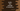# 2 ways to find the average of array numbers in kotlin## How to find the average of array numbers in Kotlin:

In this post, we will learn how to find the average value of an array of numbers in Kotlin. If we divide the sum of all numbers in an array by the total size of the array, it will give us the average value. Let’s learn how to do that in different ways in Kotlin.

### Method 1: Kotlin program to find the average of array items by iterating through the array elements:

We can iterate through the elements of an array and find the sum of all the numbers of the array.

``````fun main() {
val intNumArray = intArrayOf(1, 2, 3, 4, 5, 6, 7)
var sum = 0

for (i in intNumArray) {
sum += i
}

val avg = sum / intNumArray.size

println("Average of \${intNumArray.joinToString(",")}: \$avg")
}``````

Here,

• intNumArray is an array of integers.
• The sum variable is initialized as 0. It is used to hold the sum of all the numbers of the array.
• The for-in loop iterates through the items of the array intNumArray one by one and adds each element to sum.
• The average is calculated by dividing the sum by the size of the array. This value is assigned to the variable avg.
• The last line is printing the array elements and the calculated average value.

If you run this program, it will print the below output:

``Average of 1,2,3,4,5,6,7: 4``

### Method 2: By using the average() function:

The average() function is defined in Kotlin Iterable and it is defined as like below:

``````fun Iterable<Byte>.average(): Double

fun Iterable<Short>.average(): Double

fun Iterable<Int>.average(): Double

fun Iterable<Long>.average(): Double

fun Iterable<Float>.average(): Double

fun Iterable<Double>.average(): Double``````

Let’s change the above program to use this function.

``````fun main() {
val intNumArray = intArrayOf(1, 2, 3, 4, 5, 6, 7)

val avg = intNumArray.average()

println("Average of \${intNumArray.joinToString(",")}: \$avg")
}``````

That’s it. It will print: